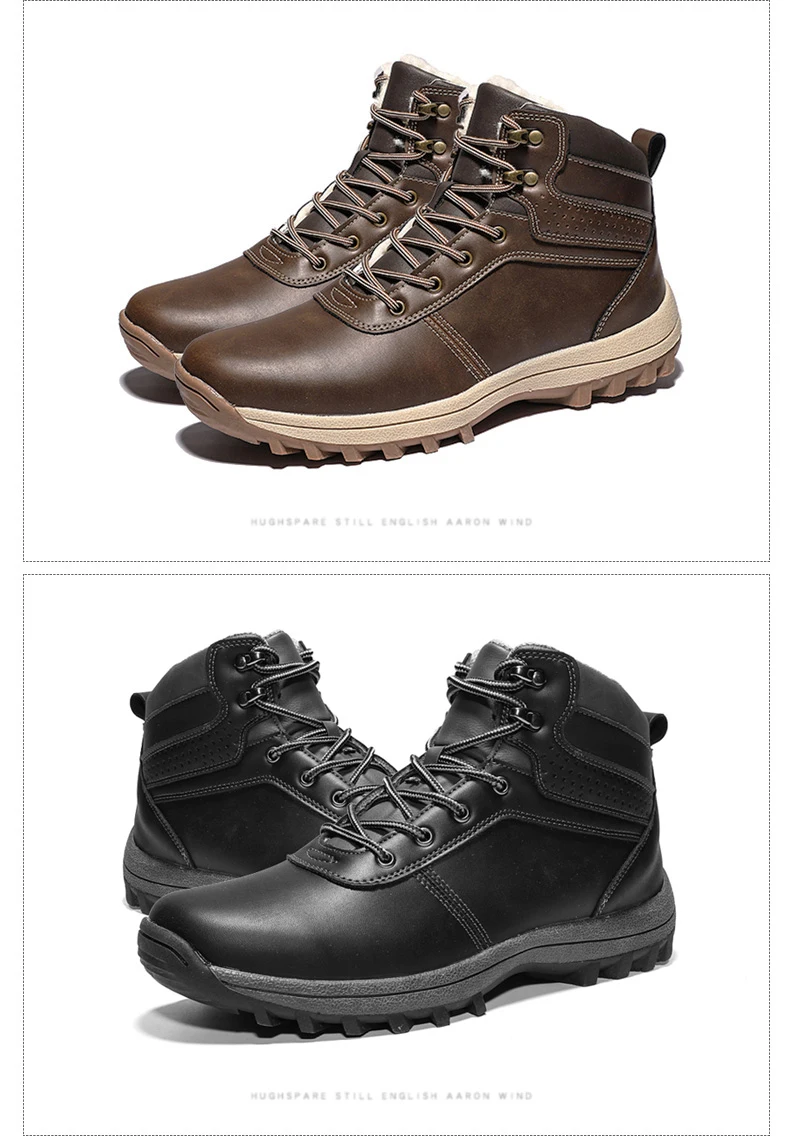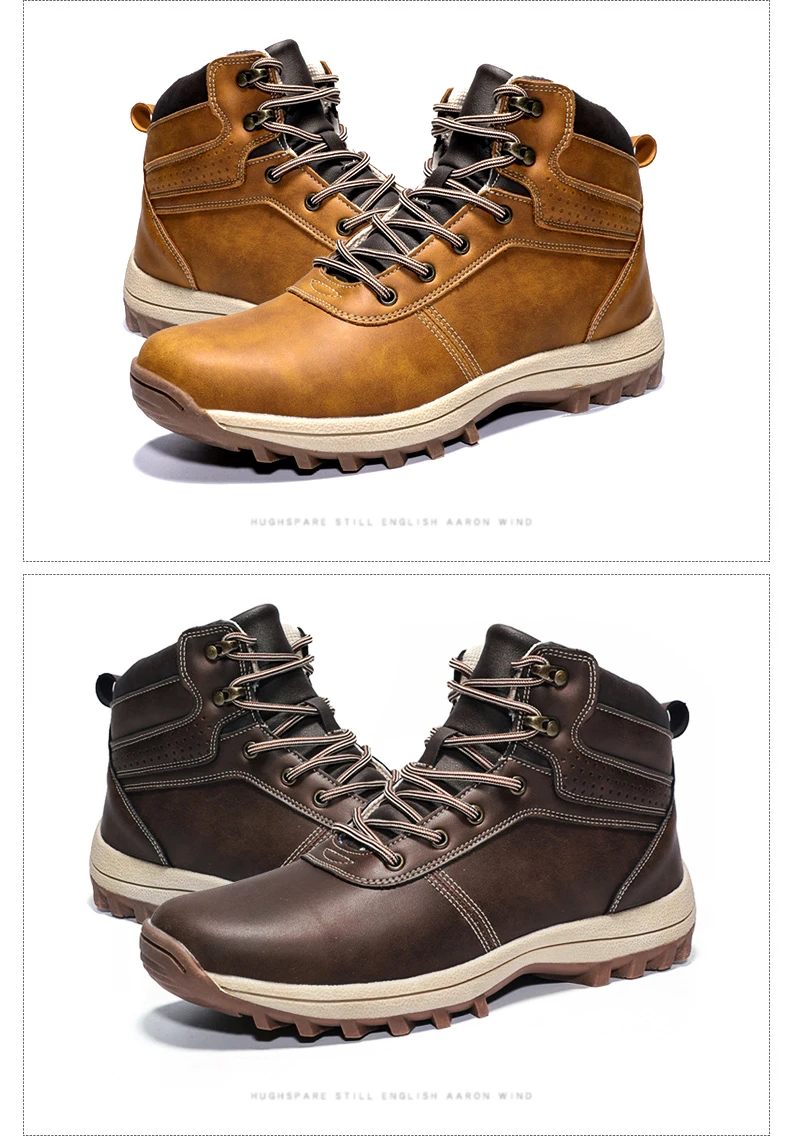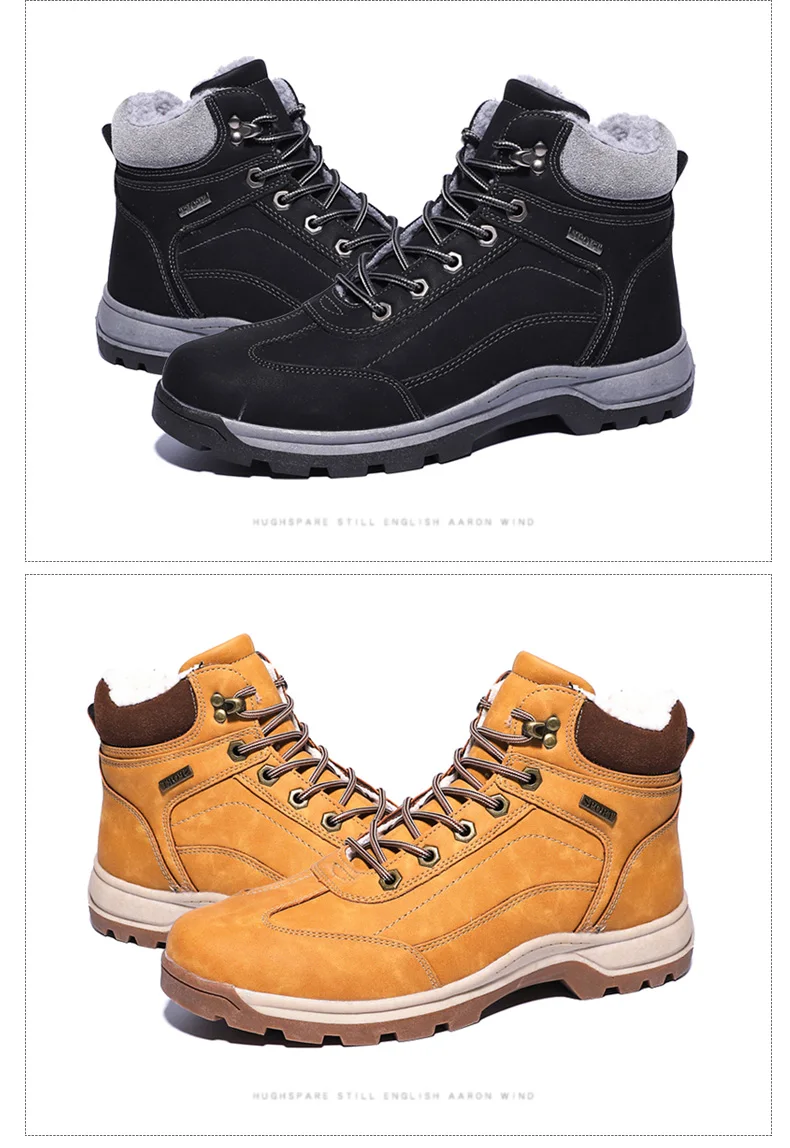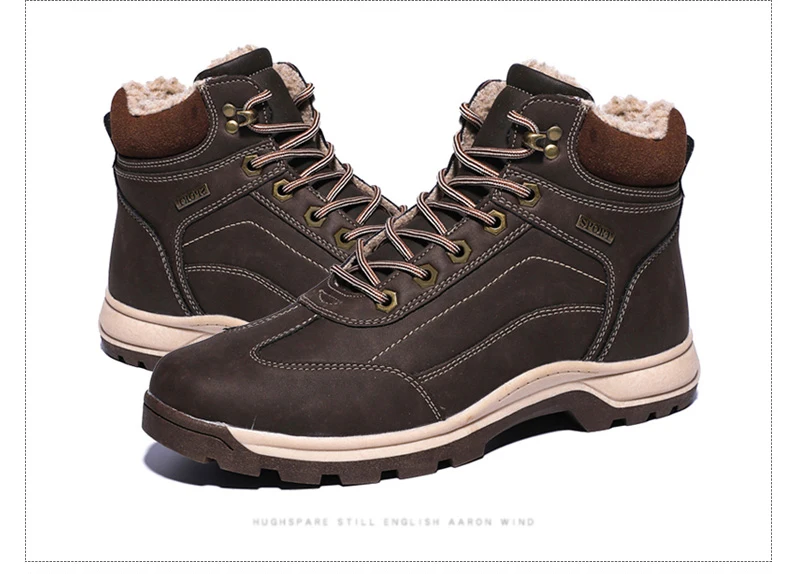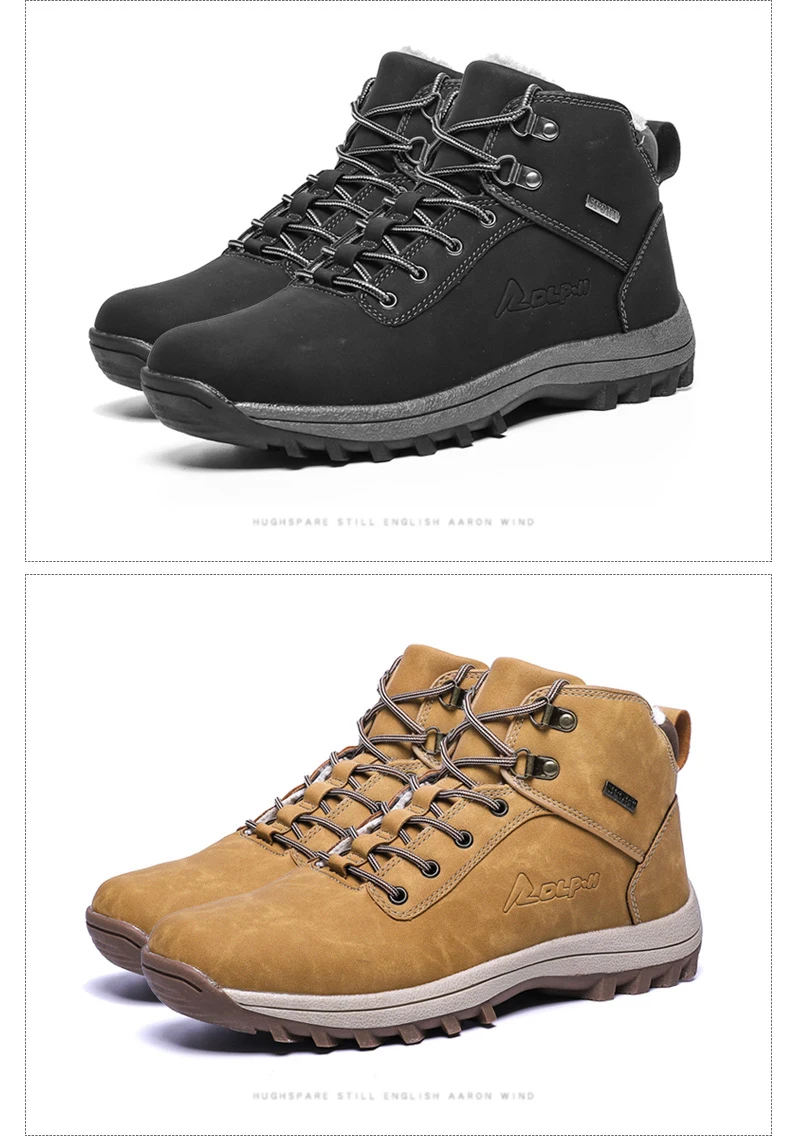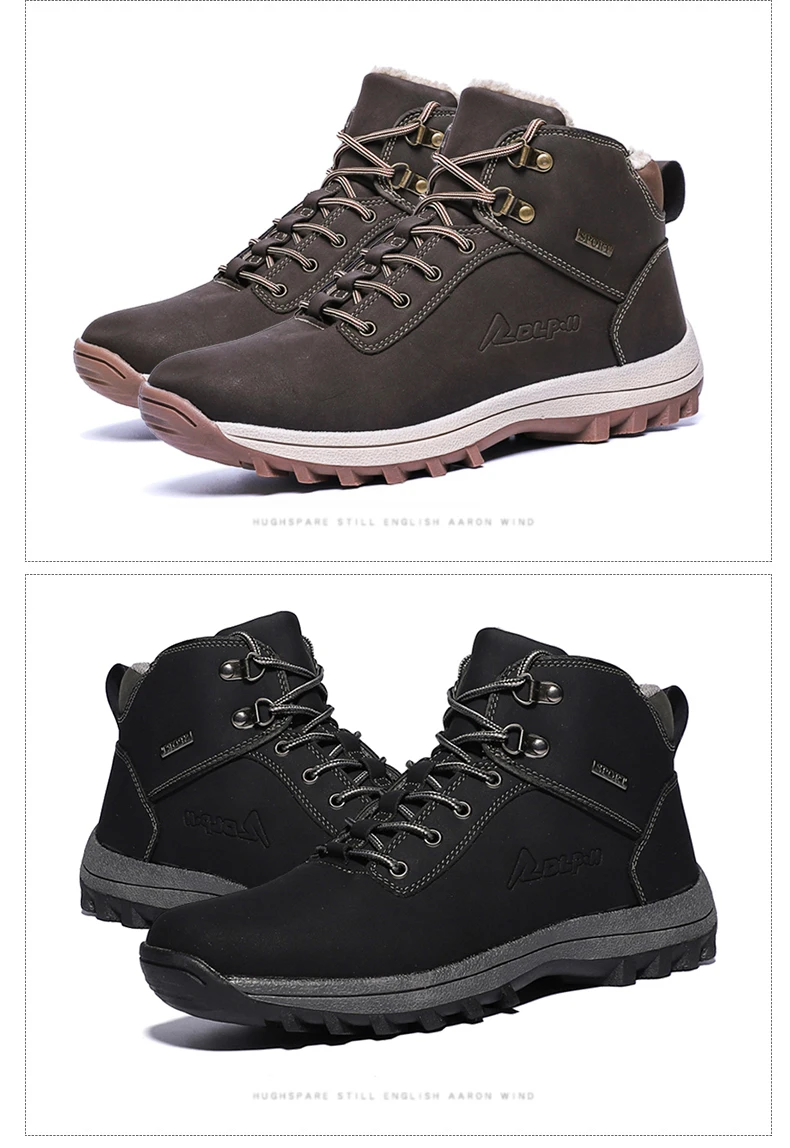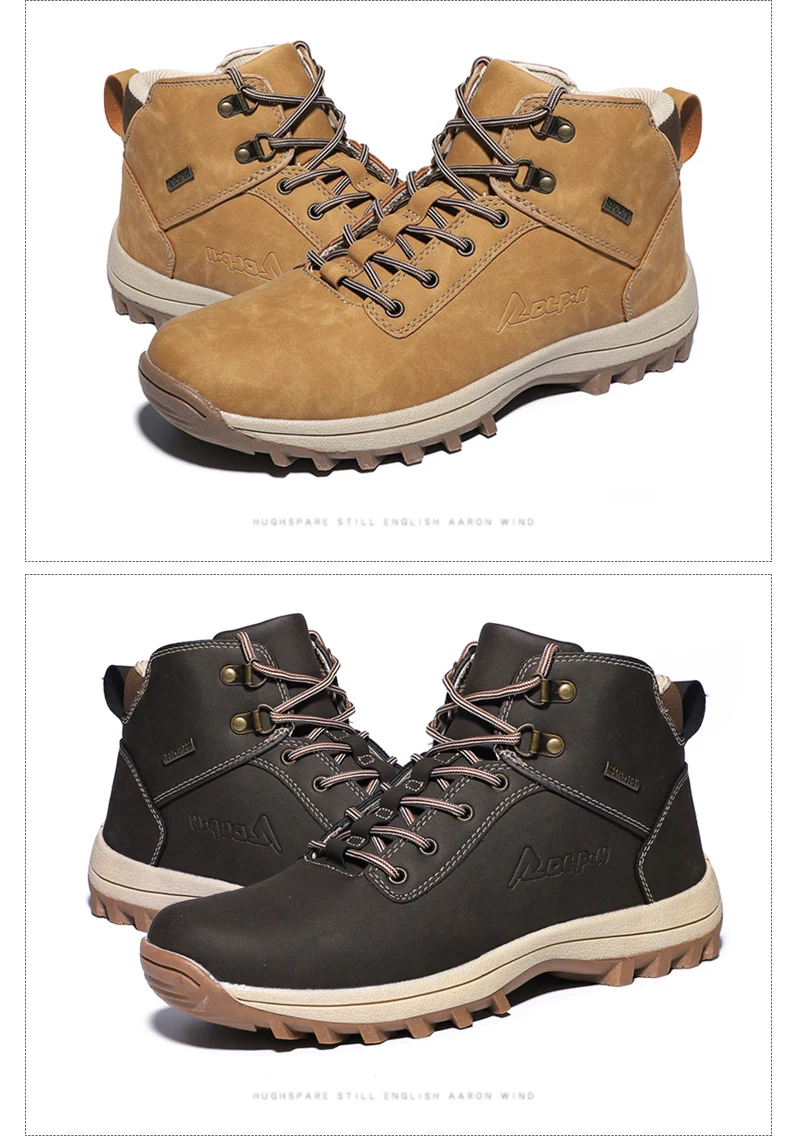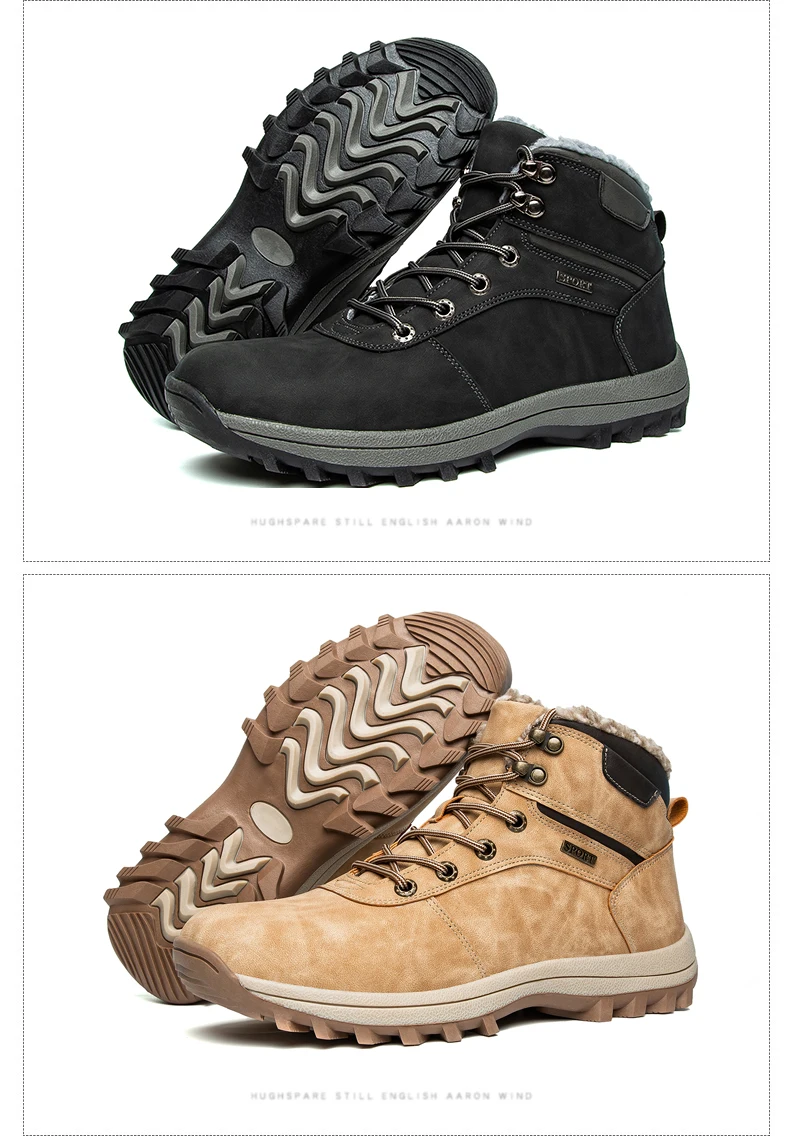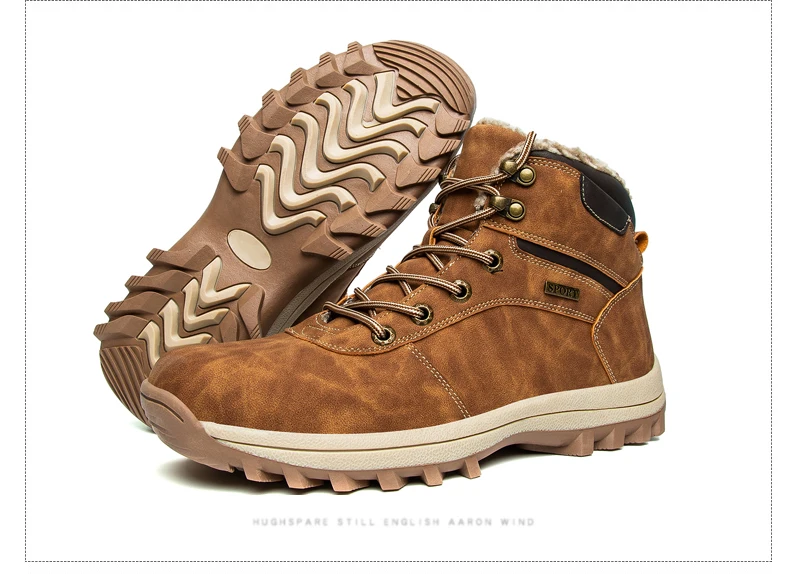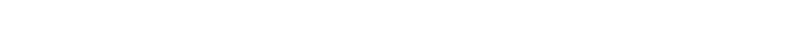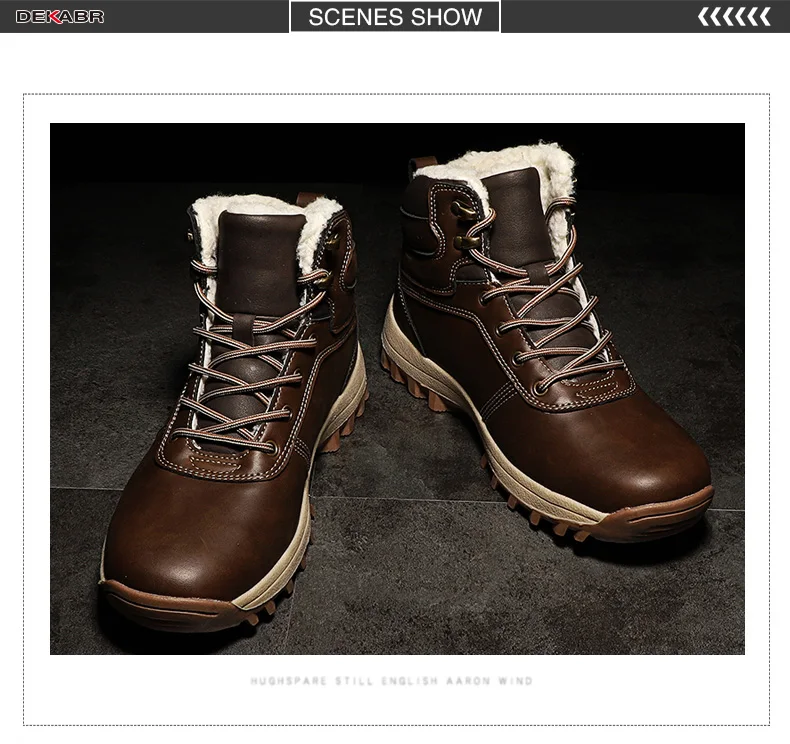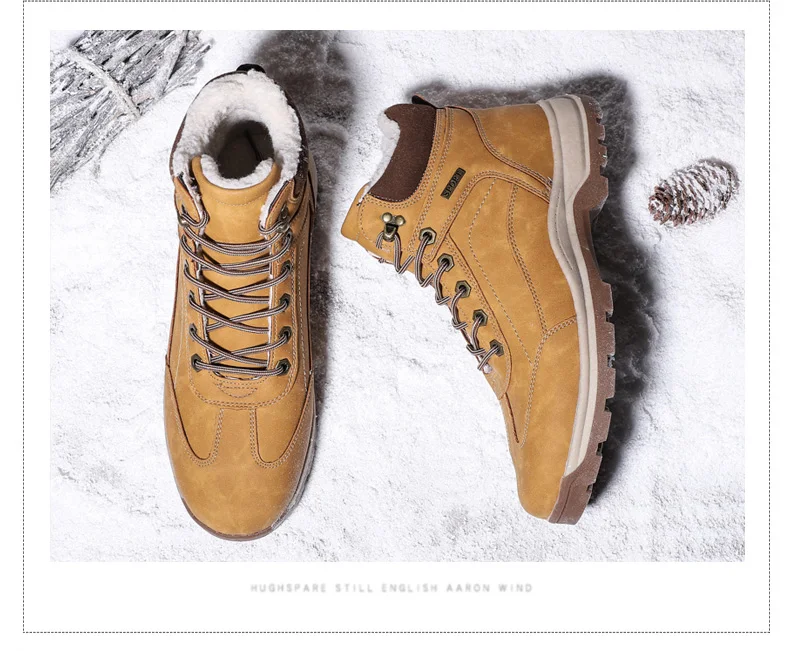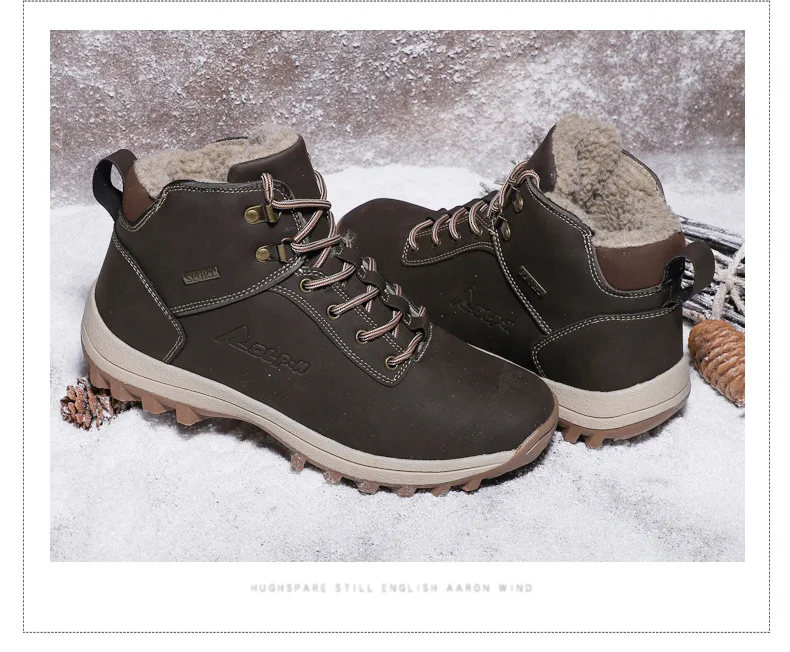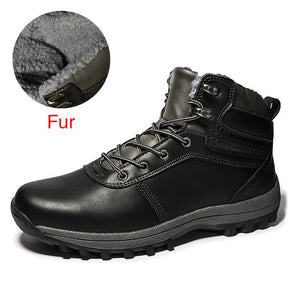# Winter Fur Warm Men Boots Genuine Leather

• \$54.98
Unit price per
• Save \$30

if your foot length (Heel to Toe) is 26.7 cm,please choose size 8.5=42 in this model.
Size 7=39      fit for foot length is 24.7cm.
Size 7.5=40   fit for foot length is 25.3cm.
Size 8=41      fit for foot length is 26cm.
Size 8.5=42   fit for foot length is 26.7cm.
Size 9.5=43   fit for foot length is 27.3cm.
Size 10=44    fit for foot length is 28cm.
Size 11=45    fit for foot length is 28.7cm
Size 12=46    fit for foot length is 29.3cm.
Size 13=47    fit for foot length is 30cm.
Size 14=48    fit for foot length is 30.6cm.